# Test: Network Theorems - 1

## 10 Questions MCQ Test Network Theory (Electric Circuits) | Test: Network Theorems - 1

Description
Attempt Test: Network Theorems - 1 | 10 questions in 30 minutes | Mock test for Electrical Engineering (EE) preparation | Free important questions MCQ to study Network Theory (Electric Circuits) for Electrical Engineering (EE) Exam | Download free PDF with solutions
QUESTION: 1

### vTH, RTH, = ?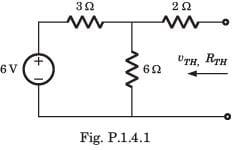Solution: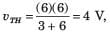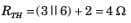QUESTION: 2

### iN, RN = ?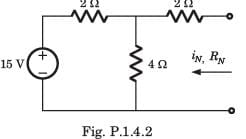Solution: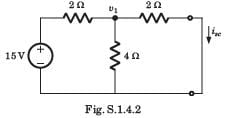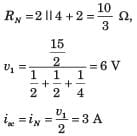QUESTION: 3

### vTH, RTH = ?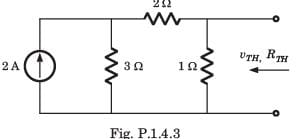Solution: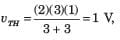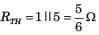QUESTION: 4

A simple equivalent circuit of the 2 terminal network shown in fig. P1.4.4 is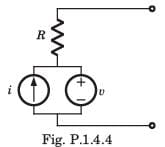Solution:

After killing all source equivalent resistance is R Open circuit voltage = v1

QUESTION: 5

iN, RN, = ?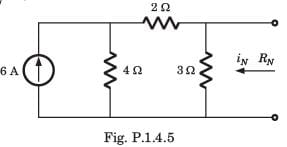Solution:

The short circuit current across the terminal is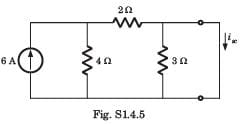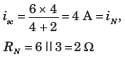QUESTION: 6

vTH, RTH = ?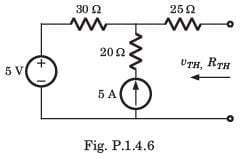Solution:

For the calculation of RTH if we kill the sources then 20 Ω resistance is inactive because 5 A source will be open circuit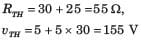QUESTION: 7

RTH = ?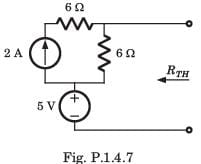Solution:

After killing the source, RTH = 6 Ω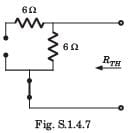QUESTION: 8

The Thevenin impedance across the terminals ab of the network shown in fig. P.1.4.8 is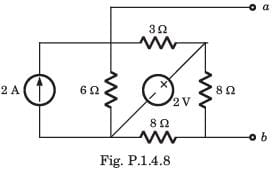Solution:

After killing all source,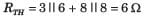QUESTION: 9

For In the the circuit shown in fig. P.1.4.9 a network and its Thevenin and Norton equivalent are given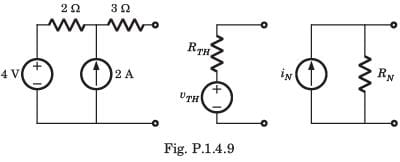The value of the parameter are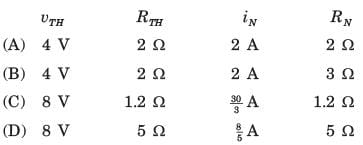Solution: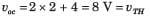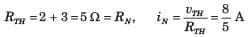QUESTION: 10

v1 = ?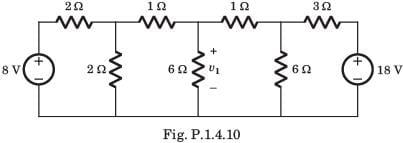Solution:

If we solve this circuit direct, we have to deal with three variable. But by simple manipulation variable can be reduced to one. By changing the LHS and RHS in Thevenin equivalent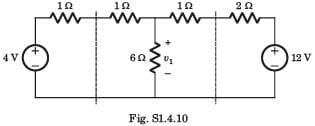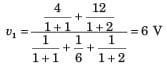Use Code STAYHOME200 and get INR 200 additional OFF Use Coupon Code